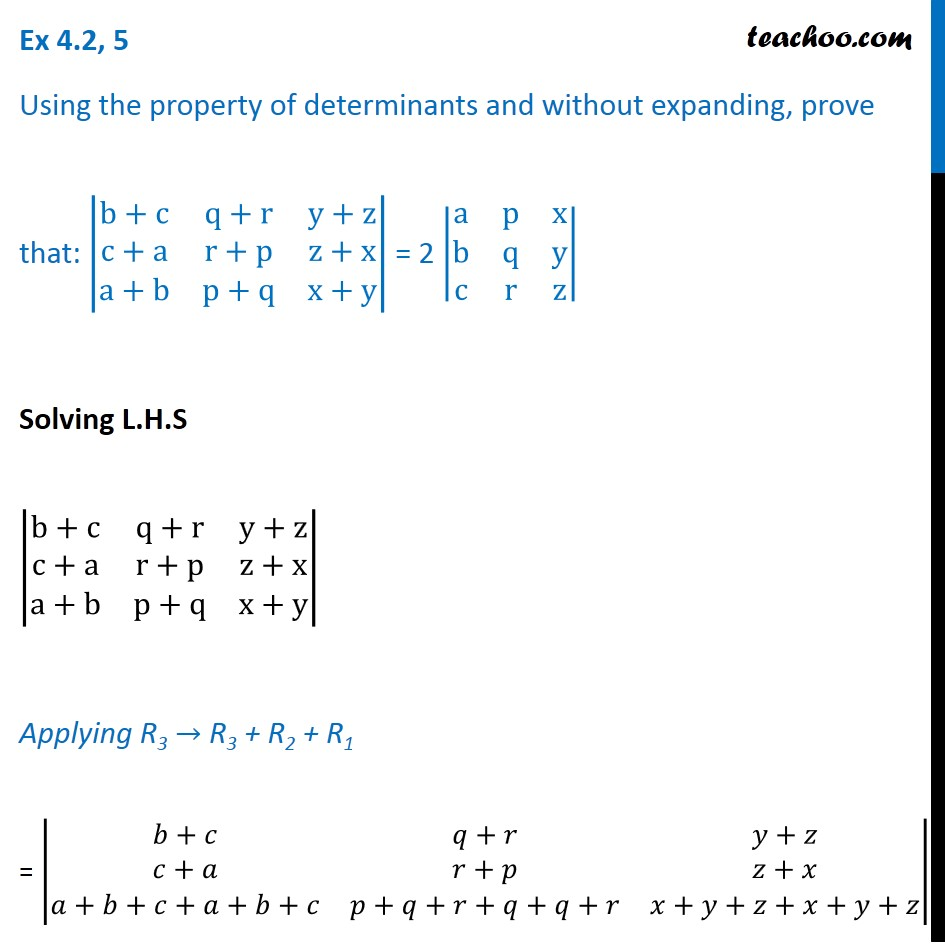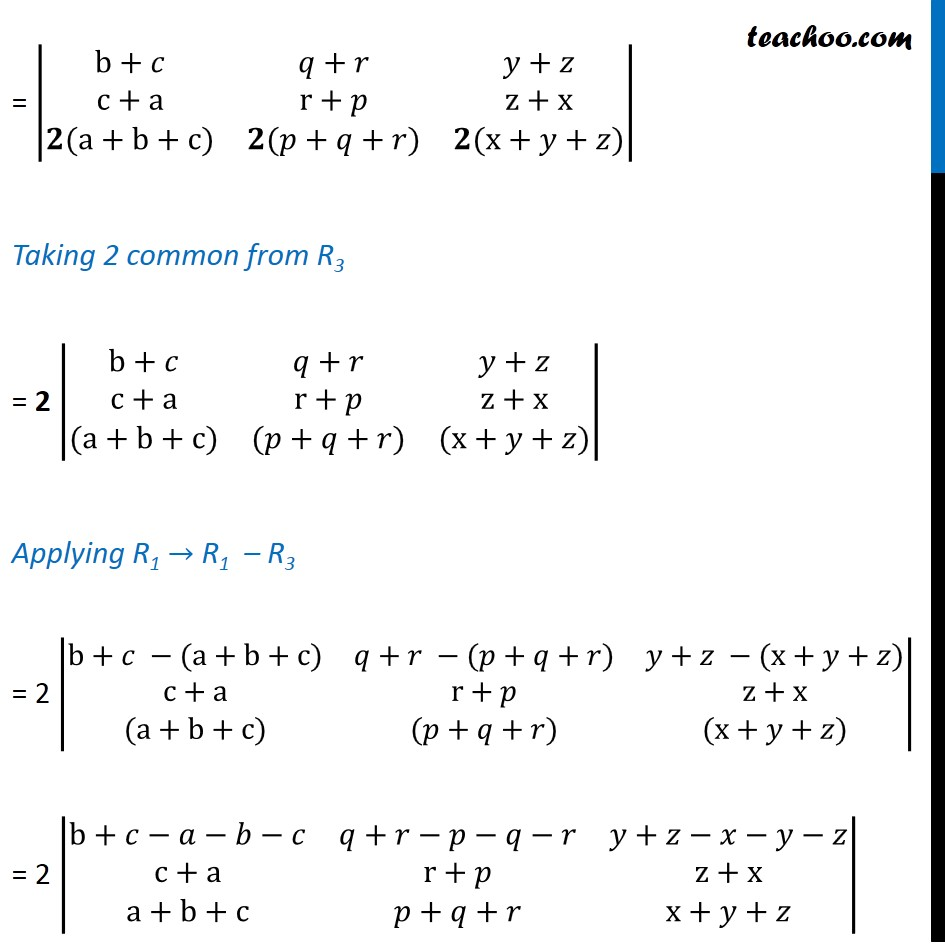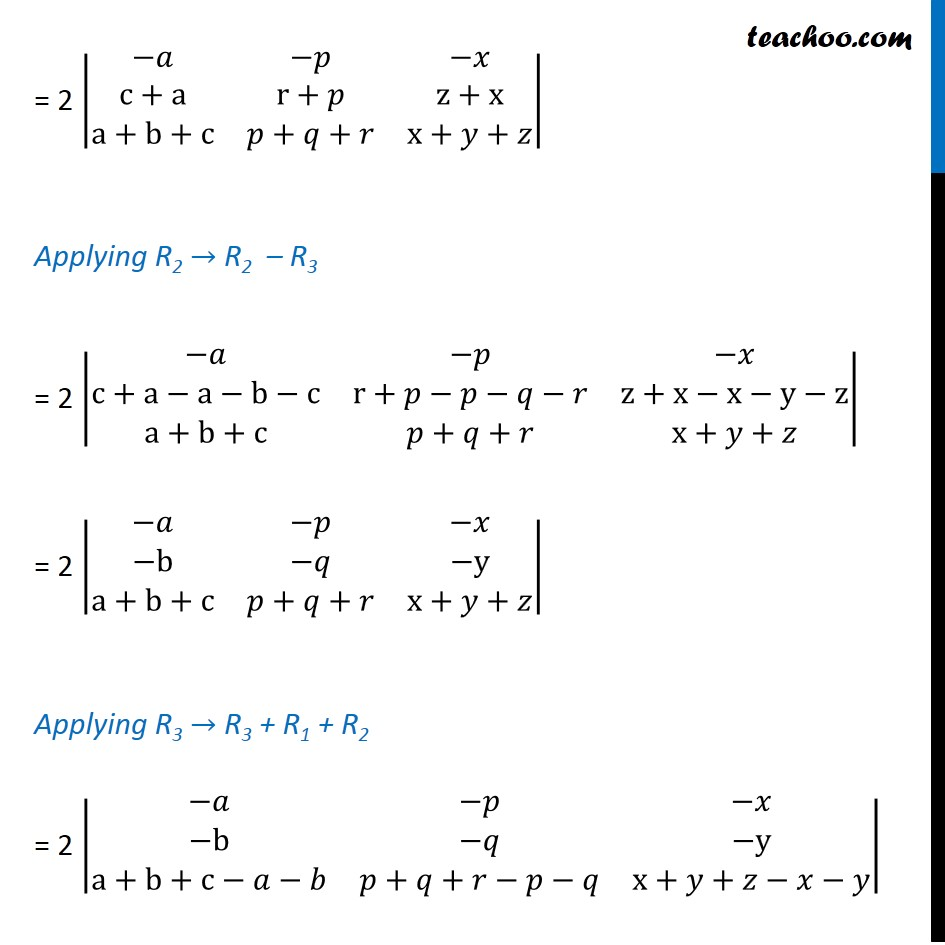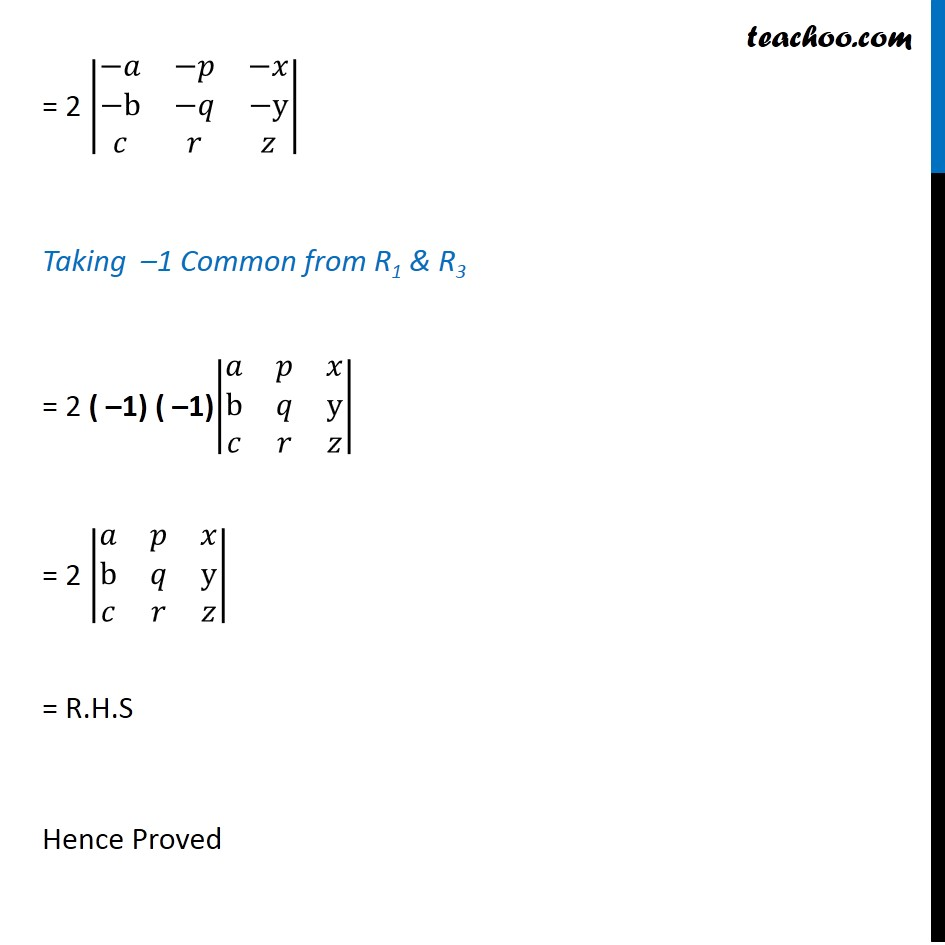Proving Determinant 1 = Determinant 2

Chapter 4 Class 12 Determinants
Concept wiseLearn in your speed, with individual attention - Teachoo Maths 1-on-1 Class

### Transcript

Question 5 Using the property of determinants and without expanding, prove that: |■8(b+c&q+r&[email protected]+a&r+p&[email protected]+b&p+q&x+y)| = 2 |■8(a&p&[email protected]&q&[email protected]&r&z)| Solving L.H.S |■8(b+c&q+r&[email protected]+a&r+p&[email protected]+b&p+q&x+y)| Applying R3 → R3 + R2 + R1 = |■8(𝑏+𝑐&𝑞+𝑟&𝑦+𝑧@𝑐+𝑎&𝑟+𝑝&𝑧+𝑥@𝑎+𝑏+𝑐+𝑎+𝑏+𝑐&𝑝+𝑞+𝑟+𝑞+𝑞+𝑟&𝑥+𝑦+𝑧+𝑥+𝑦+𝑧)| = |■8(b+𝑐&𝑞+𝑟&𝑦+𝑧@c+a&r+𝑝&z+x@𝟐(a+b+c)&𝟐(𝑝+𝑞+𝑟)&𝟐(x+𝑦+𝑧))| Taking 2 common from R3 = 2 |■8(b+𝑐&𝑞+𝑟&𝑦+𝑧@c+a&r+𝑝&z+x@(a+b+c)&(𝑝+𝑞+𝑟)&(x+𝑦+𝑧))| Applying R1 → R1 – R3 = 2 |■8(b+𝑐 −(a+b+c)&𝑞+𝑟 −(𝑝+𝑞+𝑟)&𝑦+𝑧 −(x+𝑦+𝑧)@c+a&r+𝑝&z+x@(a+b+c)&(𝑝+𝑞+𝑟)&(x+𝑦+𝑧))| = 2 |■8(b+𝑐−𝑎−𝑏−𝑐&𝑞+𝑟−𝑝−𝑞−𝑟&𝑦+𝑧−𝑥−𝑦−𝑧@c+a&r+𝑝&[email protected]+b+c&𝑝+𝑞+𝑟&x+𝑦+𝑧)| = 2 |■8(−𝑎&−𝑝&−𝑥@c+a&r+𝑝&[email protected]+b+c&𝑝+𝑞+𝑟&x+𝑦+𝑧)| Applying R2 → R2 – R3 = 2 |■8(−𝑎&−𝑝&−𝑥@c+a−a−b−c&r+𝑝−𝑝−𝑞−𝑟&z+x−x−y−[email protected]+b+c&𝑝+𝑞+𝑟&x+𝑦+𝑧)| = 2 |■8(−𝑎&−𝑝&−𝑥@−b&−𝑞&−[email protected]+b+c&𝑝+𝑞+𝑟&x+𝑦+𝑧)| Applying R3 → R3 + R1 + R2 = 2 |■8(−𝑎&−𝑝&−𝑥@−b&−𝑞&−[email protected]+b+c−𝑎−𝑏&𝑝+𝑞+𝑟−𝑝−𝑞&x+𝑦+𝑧−𝑥−𝑦)| = 2 |■8(−𝑎&−𝑝&−𝑥@−b&−𝑞&−y@𝑐&𝑟&𝑧)| Taking –1 Common from R1 & R3 = 2 ( –1) ( –1)|■8(𝑎&𝑝&𝑥@b&𝑞&y@𝑐&𝑟&𝑧)| = 2 |■8(𝑎&𝑝&𝑥@b&𝑞&y@𝑐&𝑟&𝑧)| = R.H.S Hence Proved